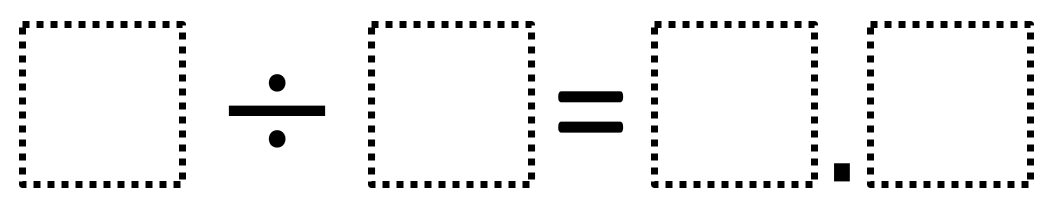Home > Grade 5 > Dividing Whole Numbers With A Decimal Quotient

# Dividing Whole Numbers With A Decimal Quotient

Directions: Using the digits 1 to 9, at most one time each, place a digit in each box to make a true statement.### Hint

How does choosing the divisor and dividend affect the quotient?
What digits would be bad choices for the divisor?
What digits would be bad choices for the dividend?

There are many possible answers including:
9 ÷ 2 = 4.5
6 ÷ 4 = 1.5
8 ÷ 5 = 1.6

Source: Owen Kaplinsky

## Multiplying Decimals to Make a Whole Number Product

Directions: Using the digits 1 to 9, at most one time each, fill in the …

1.2.